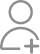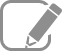Take Class 11 Tuition from the Best Tutors

•Affordable fees
•1-1 or Group class
•Flexible Timings
•Verified Tutors

Search in

# The owner of a milk store finds that, he can sell 980 litres of milk each week at Rs 14/litre and 1220 litres of milk each week at Rs 16/litre. Assuming a linear relationship between selling price and demand, how many litres could he sell weekly at Rs 17/litre?Follow 1AnswerThe relationship between selling price and demand is linear. Assuming selling price per litre along the x-axis and demand along the y-axis, we have two points i.e., (14, 980) and (16, 1220) in the XY plane that satisfy the linear relationship between selling price and demand. Therefore, the linear relationship...

The relationship between selling price and demand is linear.

Assuming selling price per litre along the x-axis and demand along the y-axis, we have two points i.e., (14, 980) and (16, 1220) in the XY plane that satisfy the linear relationship between selling price and demand.

Therefore, the linear relationship between selling price per litre and demand is the equation of the line passing through points (14, 980) and (16, 1220).When x = Rs 17/litre,Thus, the owner of the milk store could sell 1340 litres of milk weekly at Rs 17/litre.

Now ask question in any of the 1000+ Categories, and get Answers from Tutors and Trainers on UrbanPro.com

Find Class 11 Tuition near you

Looking for Class 11 Tuition ?

Learn from the Best Tutors on UrbanPro

Are you a Tutor or Training Institute?

Join UrbanPro Today to find students near you
X

### Looking for Class 11 Tuition Classes?

The best tutors for Class 11 Tuition Classes are on UrbanPro

• Select the best Tutor
• Book & Attend a Free Demo
• Pay and start Learning### Take Class 11 Tuition with the Best Tutors

The best Tutors for Class 11 Tuition Classes are on UrbanPro